### IMO Shortlist 1996 problem A6

Kvaliteta:
Avg: 0,0
Težina:
Avg: 8,0
Let$n$ be an even positive integer. Prove that there exists a positive integer$k$ such that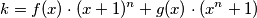for some polynomials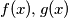$f(x), g(x)$ having integer coefficients. If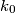$k_0$ denotes the least such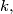$k,$ determine$k_0$ as a function of$n,$ i.e. show that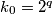$k_0 = 2^q$ where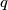$q$ is the odd integer determined by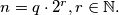$n = q \cdot 2^r, r \in \mathbb{N}.$

Note: This is variant A6' of the three variants given for this problem.
Izvor: Međunarodna matematička olimpijada, shortlist 1996